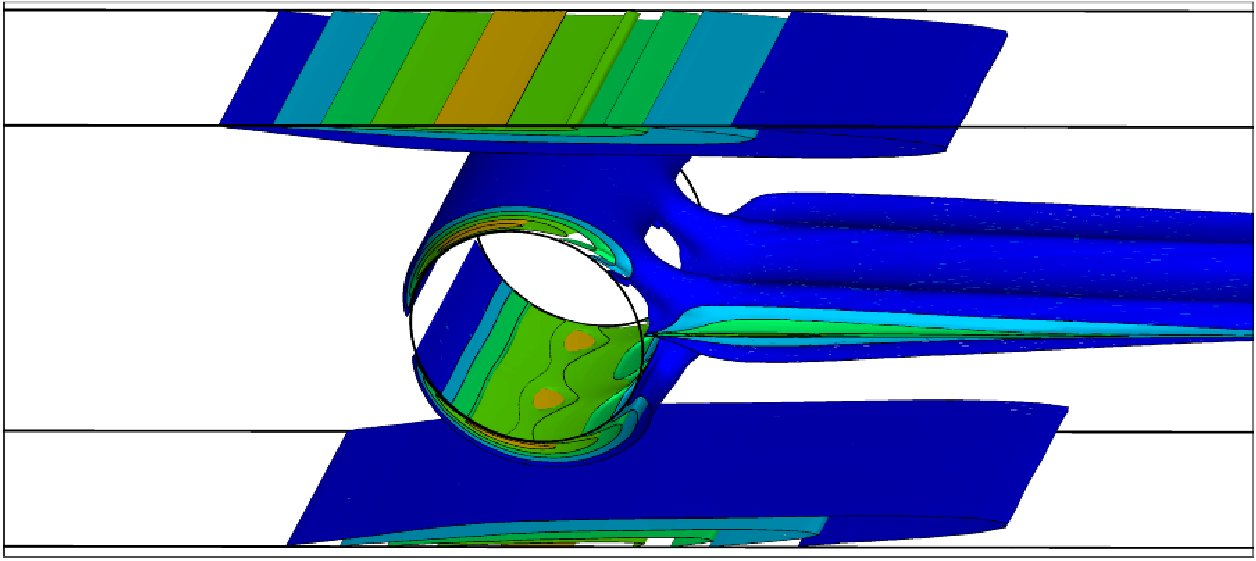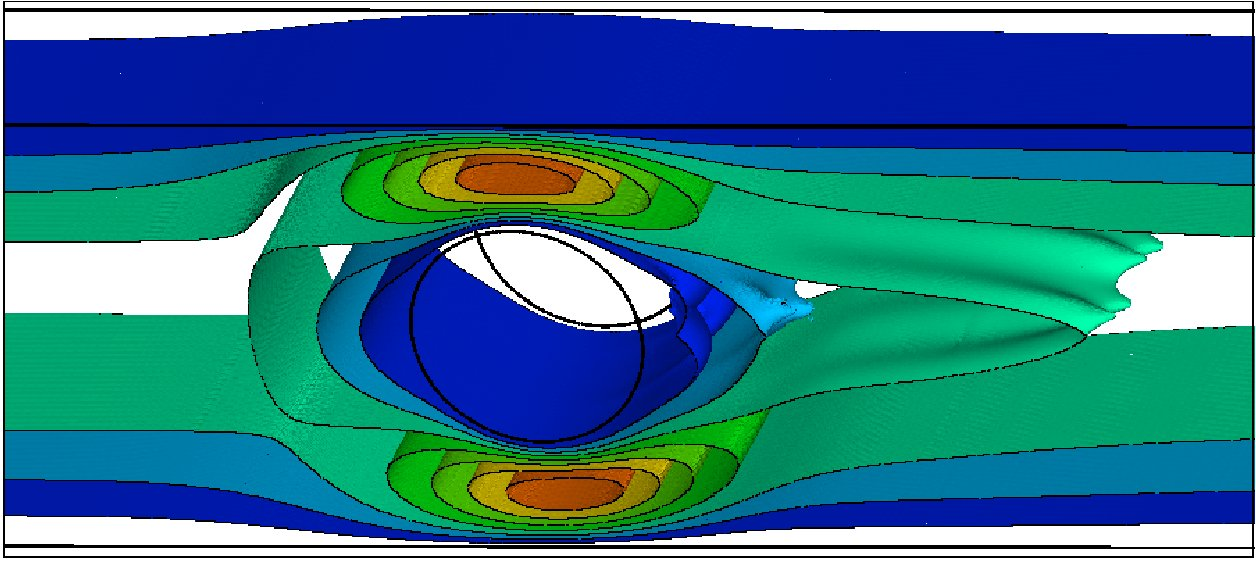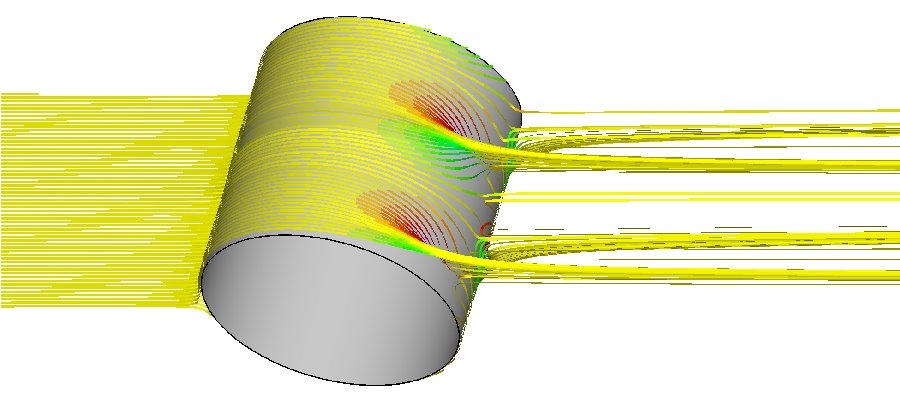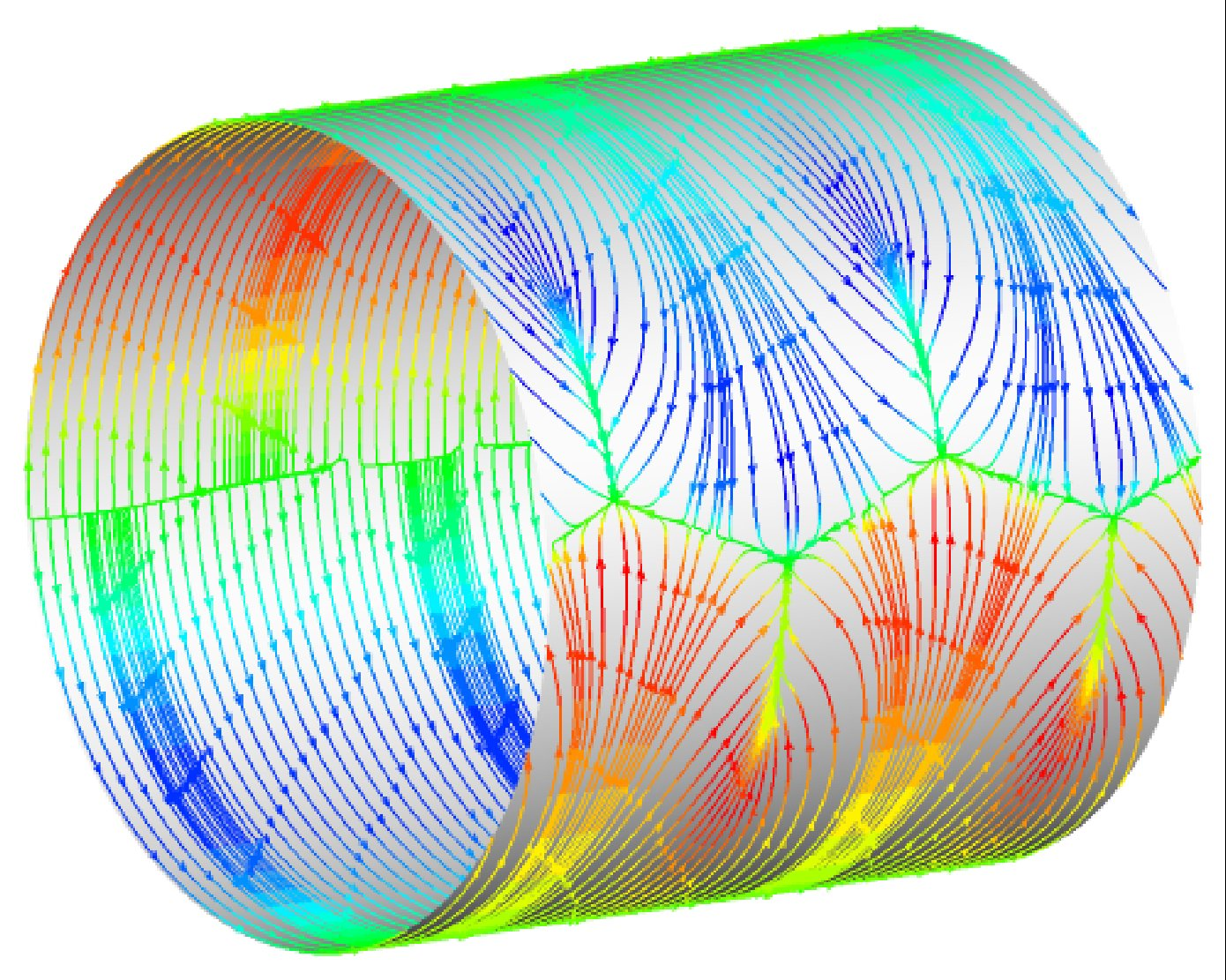VISCOELASTIC FLUID FLOW INSTABILITIES

Prediction and suppression of the onset of viscoelastic fluid flow instabilities are of great importance in many industrial polymer processing applications where output quality constraints require that operating conditions should be maintained in the stable flow regime. Therefore, the flow instabilities are the primary constraint for the processing speed in many industrial polymer forming processes such as extrusion, wire coating, blow molding, sheet-formation, etc. These viscoelastic instabilities occur in the creeping motion of non-Newtonian polymeric liquids and are entirely absent in the corresponding motion of Newtonian liquids. A parallel stable unstructured finite volume algorithm Visco-Solve has been developed in order to investigate the viscoelatic fluid flow instabilities. The numerical method is based on the side-centered finite volume method where the velocity vector components are defined at the mid-point of each cell face, while the pressure term and the extra stress tensor are defined at element centroids. The present arrangement of the primitive variables leads to a stable numerical scheme and it does not require any ad-hoc modifications in order to enhance the pressure-velocity-stress coupling. The log-conformation representation has been implemented in order improve the limiting Weissenberg numbers. The time stepping algorithm used decouples the calculation of the polymeric stress by solution of a hyperbolic constitutive equation from the evolution of the velocity and pressure fields by solution of a generalized Stokes problem. The resulting algebraic linear systems are solved using the FGMRES(m) Krylov iterative method with the restricted additive Schwarz preconditioner for the extra stress tensor and the geometric non-nested multilevel preconditioner for the Stokes system. The calculations are presented for an Oldroyd-B fluid past a confined circular cylinder in a rectangular channel at relatively high Weissenberg numbers. The present numerical calculations reveal three-dimensional purely-elastic instabilities in the wake of a confined single cylinder which is in accord with the earlier experimental results in the literature. In addition, the flow field is found out to be no longer symmetric in the wake of the cylinder at high Weissenberg numbers.The computed Txx contours around a confined circular cylinder in a channel with D/H=0.5 at We=2.0 and Re=0.0 for an Oldroyd-B fluid.The computed u-velocity contours
around a confined circular cylinder in a channel with D/H=0.5 at We=2.0 and Re=0.0 for an Oldroyd-B fluid.[a][b]
The compted streamtraces around a confined circular cylinder [a] and the surface streamlines indicating separation lines [b] at We=2.0 and Re=0.0 for an Oldroyd-B fluid
. The calculations reaveal three-dimensional viscoelastic wake instability.